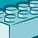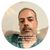# App Development

Announcements
Qlik Cloud Maintenance is scheduled between March 27-30. Visit Qlik Cloud Status page for more details.
cancel
Showing results for
Did you mean:Contributor III

## Set analysis in a pivot table

I have the following pivot table: see attached.

Rows = Month, measures

Columns = Marketing type

Measures = multiple, including "% of Loans"

% of Loans is calculated as:  that month's "Loans Generated" divided by that columns sum of "Loans Generated" across all the months.

I'm trying different versions of set analysis and total, but haven't been able to get the working formula yet.  Can someone help? Thanks!

1 Solution

Accepted SolutionsMVP

I think what you are trying should show the correct for other months, can you share sample data/app, this below one is the other alternative which I didn't put properly in the last post -

Sum(S7_Ct)

/

Also do you see any difference if you make your measures as columns and type as rows?

9 RepliesContributor III
Author

The following formula works in coming up with the column totals:  Sum( Aggr( sum(S7_Ct),S7_ADType) )

This will be the correct denominator in the "% of Loans" formula, so that looks like:

Sum(S7_Ct)

/

However ... for testing, when I put the formula in the "% of Loans" measure in the pivot table, it ONLY puts the correct column total in the month of April 2017.  For whatever reason, all the other months show up as \$0.  See attached for a screenshot of the formula in action.MVP

Can you check the result of this -

Sum(S7_Ct)

/

Sum(Total S7_Ct)

If possible please share sample data to look further.Contributor III
Author

Sum(Total S7_Ct) provides the total sum for all columns, not just the applicable column.Contributor III
Author

When I filter the pivot table on months, it seems to fill in the value for ONLY the month that comes first alphabetically.

For example, when I take out the month of April (which is the only month that had the number filled in) and only select Dec - Jan - Feb and July (randomly), only the month of December has the number completed.  The rest is \$0.

Why would only the 'first' (alphabetically) month show up with this calculation?MVP

I think what you are trying should show the correct for other months, can you share sample data/app, this below one is the other alternative which I didn't put properly in the last post -

Sum(S7_Ct)

/

Also do you see any difference if you make your measures as columns and type as rows?MVP

I think you need total by both Month and Type so, it should be like this -

It was showing other months null as it calculates once for all months, I think if you would have added nodistinct in Aggr(), it would have shown same value in all the months.Contributor III
Author

For ease of troubleshooting, I'm only placing the denominator formula (as in, this should show the total number of old loans per column).

See screenshot "pivot_table": when I use Sum(Aggr(sum(S7_Ct),Monthfield,S7_ADType)), it basically shows the count per ADType and per Month.

However, it should do the sum without looking at Month.

See screenshot "pivot_table2": without the extra aggregation on Monthfield, it does calculate correctly ... but only for the first alphabetical month!?Contributor III
Author

See attached for a sample App.  As you see, the column total only gets shown once.  I need it to show for each row, as I would expect the formula to do.Contributor III
Author

Digvijay,

I was sure I tested this formula suggestion but it does work!  Thank you!

Sum(TOTAL <Product> UnitSales)Tags
Community Browser